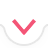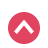# Inequalities

Quiz History

Select Topics
Basic

Moderate

Start Quiz
Quiz Assignment

00:00:00Summary
Previous Question

Show Hints

3 / 3
Sorry, your browser does not support this application
x^2
\frac{\msquare}{\msquare}
\sqrt{\square}
\nthroot[\msquare]{\square}
\infty
\log_{\msquare}
(\square,\square]
[\square,\square)
\le
\ge
\mathrm{No\:Solution}
\mathrm{True}\:\forall x
Let's Try Again :(
Try to simplify further
Thank you!

## Related Tutorials and Articles

Inequalities: Linear
Inequalities: Compound
Inequalities: Rational
Inequalities: Absolute (Part 1)
Inequalities: Logarithmic
Cheat Sheets
Start Quiz 00:00:00
Previous Question
1 / 10
x^2
\frac{\msquare}{\msquare}
\sqrt{\square}
\nthroot[\msquare]{\square}
\infty
\log_{\msquare}
(\square,\square]
[\square,\square)
\le
\ge
\mathrm{No\:Solution}
\mathrm{True}\:\forall x
Finish Quiz

Symbolab

# Summary

Submit Assignment Start Over Back

# Summary

Start Again Back
Prev Student Next Student

in 01:12:13 hoursBack
Back

## Steps

Sorry, your browser does not support this application
Edit Quiz
Quiz Saved!# Crowns

You haven't collected any crowns yet

# Quizzes